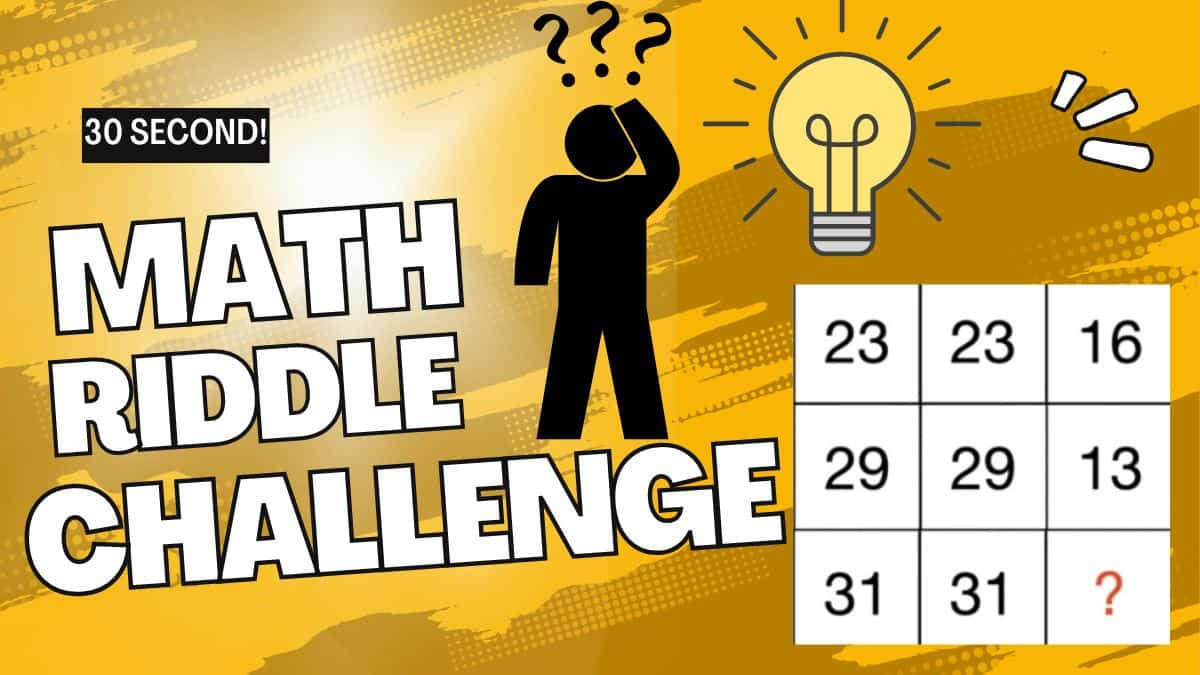# Math Riddles: Are You A Genius? Can You Solve This Square Math Puzzle Within 30 Seconds? Dare to test Your IQ Here!

Math Riddles: Can you solve these square math riddles? Only 2% of people with a high IQ can get the answer right. Dare to do this math riddle for geniuses!Math Riddles: Are You A Genius? Can You Solve This Square Math Puzzle Within 30 Seconds? Dare to test Your IQ Here!

It's Monday again, the first day of the week. What can be better than a Monday math riddle to prepare your brain for another challenging 7 days? Moreover,  Math riddles are an integral part of recreational mathematics. They do not involve any kind of pressuring competition, just you and your fun math puzzle!

The solver is supposed to find the solution and interact with the math puzzle in a logical manner to find the correct answer.

Can you do it? Strategizing the moves and applications can be difficult but not scary. In addition, these math riddles and puzzles sharpen your brain to a great extent.

## What are Math riddles?

Math Riddles are puzzle games where players try to figure out a solution to a problem. As fun as they are, the practice also challenges your brain. They are a great way to boost mental capacity and lateral thinking skills, maintain memory strength, and delight you! It often requires thinking unconventionally with given constraints in mind; sometimes, it involves lateral thinking.

Let’s find out if all of this is true or not! Are you ready?

Here we go!

## Math Riddle Challenge

Look at the picture posted below. You will find a math puzzle that only those with a high IQ will be able to answer. Carefully check this math puzzle and find the missing number.

source: simplylogical

Is it easy?

Were you able to solve this tricky math puzzle?

If you were able to solve it, let's make it a challenge.

Dare to do it in 30 seconds!

Find out how fast you can solve this math puzzle. If you can solve this puzzle in less time, then that's even better.

Now, go ahead and bring out your mathematical brain to solve this tricky puzzle. Your time starts now.

All the best!

Tick…

Tock…

Tick..

Time’s Up!

 Math fact! The numbers on opposite sides of a die always add up to seven.

Have you solved the square math puzzle yet? We are going to reveal the answers now. Let’s solve it together.

## Solution

To solve a mathematical puzzle, observe the puzzle and try to solve it to find the solution to the problem. If you cannot find the solution, we have an answer for you. If you are still working to figure it out, we have the solution to this mathematical puzzle. This puzzle will allow you to see how keen you are and how reasonable an observer you are. If you still need help, the picture below will help you understand the solution.

Since you have gone through the image, there is a chance that you have some idea about the puzzle we have given. If you are still going through the puzzle, no worries; take your time, and there is a chance for you to find the solution for the image. So, make your brain get some ideas about the puzzle given and use your mathematics skills to their full extent to solve this problem.

Here is the Solution

The square math puzzle can be divided into three equations to find the missing number

## Equation 1

Here three squares are given

### 5+2+9=16

So by this logic if we multiply the first two numbers of each row we get the third square number on adding all the constants of the product.

Similarly we can try the next row,

## Final Equation

### 31,31,?

Let's find the missing number

## The solution is 16

Great work!

Don't miss out!

Math Riddles: Do You Have A High IQ? Solve These Figures and Find the Missing Number!

Get the latest General Knowledge and Current Affairs from all over India and world for all competitive exams.
खेलें हर किस्म के रोमांच से भरपूर गेम्स सिर्फ़ जागरण प्ले पर